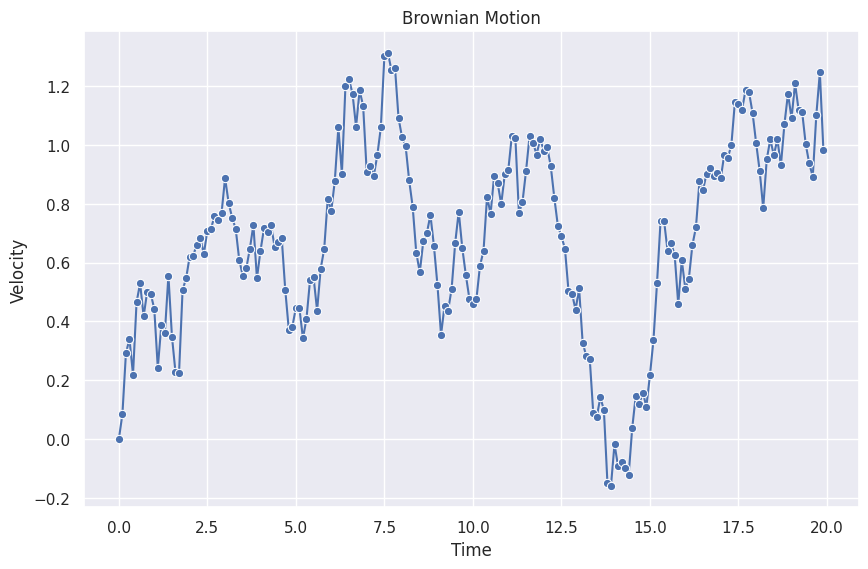# Time Series Data Generating Process: Langevin Equation¶

Among the many data generating processes (DGP), the Langevin equation is one of the most interesting DGP.

## Brownian Motion¶

Brownian motion as a very simple stochastic process can be described by the Langevin equation1. In this section, we simulate a time series dataset from Brownian motion.

Macroscopically, Brownian Motion can be described by the notion of random forces on the particles,

$\frac{d}{dt} v(t) + \gamma v(t) = R(t),$

where $$v(t)$$ is the velocity at time $$t$$ and $$R(t)$$ is the stochastic force density from the reservoir particles. Solving the equation, we get

$v(t) = v(0)e^{-\gamma t} + \int_0^t dt' e^{-\gamma (t-t')} R(t') .$

To generate a dataset numerically, we discretize it by replacing the integral with a sum,

$v(t) = v(0) e^{-\gamma t} + \sum_{n=0}^N \Delta t e^{-\gamma (t - t_n)} R(t_n)$

where $$t_i = i * \Delta t$$ and $$t = t_n$$, thus the equation is further simplified,

$v(N\Delta t) = v(0) e^{-\gamma N\Delta t} + \sum_{n=0}^N e^{-\gamma (N - n)\Delta t} R(n\Delta t) \Delta t.$

The first term in the solution is responsible for the exponential decay and the second term calculates the effect of the stochastic force.

To simulate a Brownian motion, we can either use the formal solution or the differential equation itself. Here we choose to use the differential equation itself. To simulate the process numerically, we rewrite

$\frac{d}{dt} v(t) + \gamma v(t) = R(t),$

as

$\Delta v (t+1) = R(t) \Delta t - \gamma v(t) \Delta t.$

The following is a simulated 1D Brownian motion.We create a stepper to calculate the next steps.

import numpy as np
import copy
import matplotlib.pyplot as plt
import seaborn as sns; sns.set()

## Define Brownian Motion
class GaussianForce:
"""A Gaussian stochastic force iterator.
Each iteration returns a single sample from the corresponding
Gaussian distribution.

:param mu: mean of the Gaussian distribution
:param std: standard deviation of the Gaussian distribution
:param seed: seed for the random generator
"""

def __init__(self, mu: float, std: float, seed: Optional[float] = None):
self.mu = mu
self.std = std
self.rng = np.random.default_rng(seed=seed)

def __next__(self) -> float:
return self.rng.normal(self.mu, self.std)

class BrownianMotionStepper:
"""Calculates the next step in a brownian motion.

:param gamma: the damping factor $\gamma$ of the Brownian motion.
:param delta_t: the minimum time step $\Delta t$.
:param force_densities: the stochastic force densities, e.g. [GaussianForce][eerily.data.generators.brownian.GaussianForce].
:param initial_state: the initial velocity $v(0)$.
"""

def __init__(
self,
gamma: float,
delta_t: float,
force_densities: Iterator,
initial_state: Dict[str, float],
):
self.gamma = gamma
self.delta_t = delta_t
self.forece_densities = copy.deepcopy(force_densities)
self.current_state = copy.deepcopy(initial_state)

def __iter__(self):
return self

def __next__(self) -> Dict[str, float]:

force_density = next(self.forece_densities)
v_current = self.current_state["v"]

v_next = v_current + force_density * self.delta_t - self.gamma * v_current * self.delta_t

self.current_state["force_density"] = force_density
self.current_state["v"] = v_next

return copy.deepcopy(self.current_state)

## Generating time series
delta_t = 0.1
stepper = BrownianMotionStepper(
gamma=0,
delta_t=delta_t,
force_densities=GaussianForece(mu=0, std=1),
initial_state={"v": 0},
)

length = 200

history = []
for _ in range(length):
history.append(next(stepper))

df = pd.DataFrame(history)

fig, ax = plt.subplots(figsize=(10, 6.18))
sns.lineplot(
x=np.linspace(0, length-1, length) * delta_t,
y=df.v,
ax=ax,
marker="o",
)

ax.set_title("Brownian Motion")
ax.set_xlabel("Time")
ax.set_ylabel("Velocity")


1. Ma L. Brownian Motion — Statistical Physics Notes. In: Statistical Physics [Internet]. [cited 17 Nov 2022]. Available: https://statisticalphysics.leima.is/nonequilibrium/brownian-motion.html

Contributors: LM